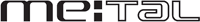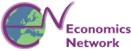# Film Series Four: Linear Programming

In the first part of this programme we illustrate how two businesses can make decisions that help maximise profits as a result of using the mathematical technique of of linear programming.

These video clips and animations can be viewed in isolation, or you can use our learning pathway and follow links to related material in our Question Bank.

 1 Watch or downloadthe video & animation 2  View related question material

#### 4.01 Introduction to Linear Programming

This segment introduces the film series and examines how two businesses can make decisions that help maximise profits as a result of using the mathematical technique of linear programming. Belgian chocolates are famous the world over. But there are many different kinds of chocolate that can be made. How can the producer pick the best combinations within the various constraints imposed on the business? We see how the mathematics of linear programming can help to give an insight into this question. (10 minutes 55 seconds).

#### 4.02 Linear Programming - Final Two Steps

In the previous section we established what is possible if our chocolatier is to meet the constraints imposed on his business. How do we find the best combination that produces the maximum profit. We now follow the final two steps in linear programing. (9 minutes 14 seconds).

#### 4.03 Linear Programming - Conclusion

Summary of the value of linear programming in business decision making. (29 seconds).

#### 4.04 Linear Programming - Tomato Farmer (part one)

Hugh and his partners at Wight Salads grow tomatoes exclusively. Although they grow many varieties, tomatoes are all that they produce for leading supermarket chains in the UK. Should they diversify into related products such as lettuce? Linear Programming helps them to arrive at an answer. (12 minutes 36 seconds).

Erratum: About two minutes from the end, the audio narration swaps T and L in part of the explanation. The inequalities as they are written on the screen are correct. We apologise for this mistake.

#### 4.05 Linear Programming - Tomato Farmer (part two)

Using linear programming we now take the last two steps to arrive at an answer about whether this tomato farmer should diversify into lettuce production. (4 minutes 4 seconds).

#### 4.06 Non-Linear Functions - Introduction

The mathematics of Linear Programming is a useful tool. However, many relationships are not linear. How do we establish the price and quantity of a commodity if supply and demand functions are non-linear? Similarly, there may be non-linear relationships in the market for labour. And as we shall see, even where a demand curve is linear some of the important relationships that exist within such markets may not be linear. (10 minutes 27 seconds).

#### 4.07 Non-Linear Functions - Labour Markets

If a labour market has both non-linear supply and demand curves, how can we find the equilibrium wage rate and quantity of labour supplied? This segment looks at examples of non-linear supply and demand curves in several industries. (9 minutes 3 seconds).

#### 4.08 Introduction to Calculus

Now we extend the analysis of non-linear functions by beginning to illustrate the use of differential calculus. This is an essential tool for economists because in many areas of economics we are interested in the speed of change of functions as a means of dealing with economic problems. We will look at two simple examples here and develop further examples in our final film to extend our understanding of differential calculus. (6 minutes 20 seconds).

#### 4.09 Calculus - Determining Marginal Revenue

This film examines the calculations for deriving marginal and total revenue with the linear demand curve of a firm with some monopoly power, and we calculate the effect on revenue of a change in output. (5 minutes 37 seconds).

#### 4.10 Calculus - Income Growth, Consumption and Savings

Slovenia recently joined the EU and is experiencing sustained growth in income. If it succeeds in growing at an annual rate of 4 per cent for the next two years average income will be rather higher. But how will that growth in income affect consumption and saving? We use Differential Calculus to find out. (5 minutes and 44 seconds).